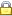DIPARTIMENTO DI INGEGNERIA DELL'INFORMAZIONEE SCIENZE MATEMATICHE UNIVERSITA' DEGLI STUDI DI SIENAPrivacy e Cookie policy

 Research Areas

 Mathematical Analysis
The Mathematical Analysis Group carries out research on a wide range of problems in the area of ordinary and partial differential equations, dynamical systems and the analytic aspects of convexity with particular emphasis on their role in the applications. Many of the problems concerning differential equations and dynamical systems pertain to the existence and the qualitative properties of solutions of autonomous and non-autonomous differential equations. To deal with these problems such equations are studied using tools of nonlinear functional analysis such as fixed point and topological degree theory as well as the theory of ordinary and partial differential equations. Particular classes of dynamical systems with structural and input discontinuities are modelled by differential inclusions, they are studied by means of tools of the multi-valued and non-smooth analysis.

Inequalities of isoperimetric type are also studied and applied to describe geometric properties of solutions of PDE's, in the framework of the Brunn-Minkowski theory for convex bodies. Methods and results from that theory are employed for solving problems in geometric tomography.

Some specific themes that have been taken up by the Mathematical Analysis Group in the last few years and which are still under active study are the following.

1. Oscillations in perturbed dynamical systems.

2. Dynamical analysis of Cellular Neural Networks.

3. Stability and control of systems governed by evolution equations.

4. Analytic aspects of convexity: theory and applications.

People
Full Professors: Giovanni Bellettini

 1.Oscillations in perturbed dinamical systems 2.Stability and control of evolution equations 3.Dynamical analysis of Cellular Neural Networks 4.Analytic aspects of the convexity: theory and applications BibliographyUsername: Password:

 Dipartimento di Ingegneria dell'Informazione e Scienze Matematiche - Via Roma, 56 53100 SIENA - Italy